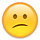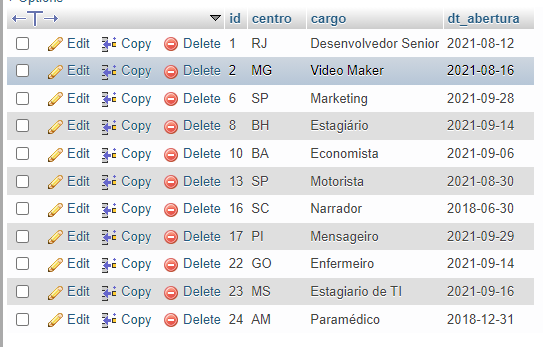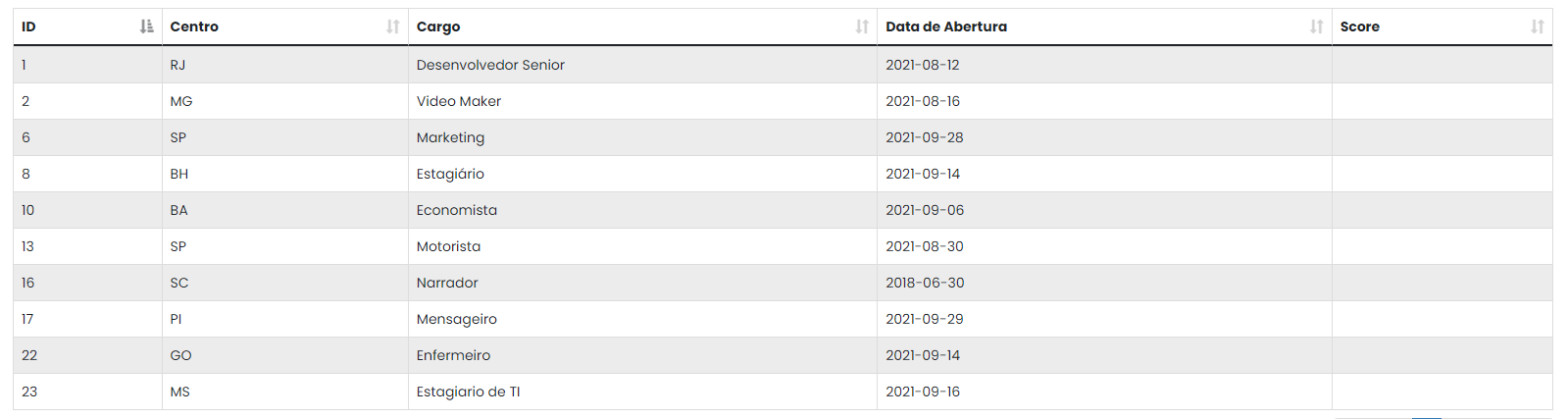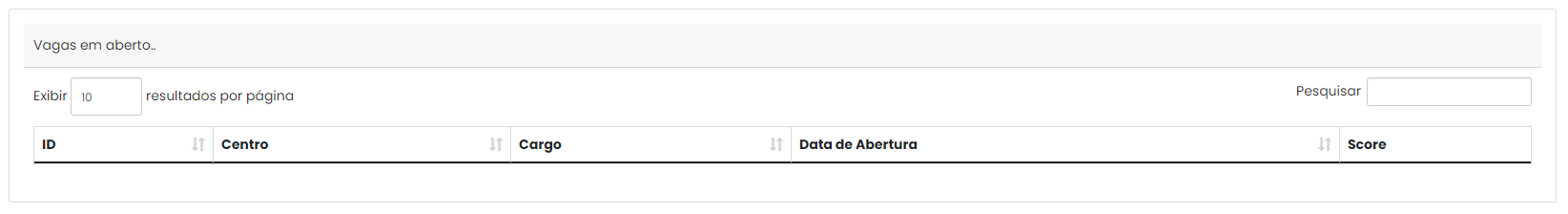# How could I add a new column when reading my data from a database?

Description of problem:
Hey guys. I'm trying to create a table with a new column that doesn't exist in my original table. I can only insert the data directly into the table after it's generated, but that way I can't reorder the table...(or I'm probably doing something wrong.) What do you guys suggest me to do?

My original table:The table that I would like to create:***The extra column on the far right

I've even thought about creating a temporary table with the additional column and the data I want to insert, but I had a problem with the syntax execution, so that's why I'm asking for your help.

Description of problem deeply:
The extra column I want to create will create a 'score system' checking the other columns (except ID column, of course). So, for example, if column 1 ('center') contains the word 'SP', the value to be stored in column 'score' will be 5; otherwise, the value will be 0. And so on.

That way, I would add all these conditions, column by column, and create a score; ordered from highest value to lowest value.

JavaScript Code:

``````    \$(document).ready(function() {
var table = \$('#tabela').DataTable({
"language": {
"url": "//cdn.datatables.net/plug-ins/1.11.1/i18n/pt_br.json"
},
"processing": true,
"serverSide": true,
"order": [
[0, "asc"]
],
"ajax": {
url: "fetch.php",
type: "POST"
}
});

function _(element) {
return document.getElementById(element);
}

\$('#tabela tbody').on('click', 'tr', function() {
var data = table.row(this).data();
alert('Você clicou na vaga "' + data + '", do centro "' + data + '" de ID: ' + data);
});

\$(".sidebar-btn").click(function() {
\$(".wrapper").toggleClass("collapse");
});
});
``````

PHP:

``````    <?php
include('./database_connection.php');

\$column = array("id", "centro", "cargo", "dt_abertura");

\$query = "SELECT * FROM vagas";

if (isset(\$_POST["search"]["value"])) {
\$query .= '
WHERE centro LIKE "%' . \$_POST["search"]["value"] . '%"
OR cargo LIKE "%' . \$_POST["search"]["value"] . '%"
OR dt_abertura LIKE "%' . \$_POST["search"]["value"] . '%"
';
}

if (isset(\$_POST["order"])) {
\$query .= 'ORDER BY ' . \$column[\$_POST['order']['0']['column']] . ' ' . \$_POST['order']['0']['dir'] . ' ';
} else {
\$query .= 'ORDER BY id DESC ';
}
\$query1 = '';

if (\$_POST["length"] != -1) {
\$query1 = 'LIMIT ' . \$_POST['start'] . ', ' . \$_POST['length'];
}

\$statement = \$connect->prepare(\$query);

\$statement->execute();

\$number_filter_row = \$statement->rowCount();

\$statement = \$connect->prepare(\$query . \$query1);

\$statement->execute();

\$result = \$statement->fetchAll();

\$data = array();

foreach (\$result as \$row) {
\$sub_array = array();
\$sub_array[] = \$row['id'];
\$sub_array[] = \$row['centro'];
\$sub_array[] = \$row['cargo'];
\$sub_array[] = \$row['dt_abertura'];
\$data[] = \$sub_array;
}

function count_all_data(\$connect)
{
\$query = "SELECT * FROM vagas";
\$statement = \$connect->prepare(\$query);
\$statement->execute();
return \$statement->rowCount();
}

\$output = array(
'draw'   => intval(\$_POST['draw']),
'recordsTotal' => count_all_data(\$connect),
'recordsFiltered' => \$number_filter_row,
'data'   => \$data
);

echo json_encode(\$output);
``````

Any help will be appreciated!

## Replies

• Sounds like `columns.render` is what you want to use. See this example of how to access the row data to render "calculated" data into one column.

Kevin

For the editing components, Editor can do that - see example here,

Colin

• edited September 11

@kthorngren Thanks, the column render solution worked perfectly. Appreciated!
But, for now, I am getting a new error:

When I am trying to initialize my table based on the new column ('score'), the table isn't loading correctly:JavaScript:

``````"columnDefs": [
{
"data" : null,
"render": function ( data, type, row ) {
if(row == 'SP'){
return 5;
}
else{
return 1;
}

},
"targets": 4,
"orderable": true,
}
],
"order": [[4, "desc"]],
``````

I think it's because my \$query function isn't "align" with the new column.

PHP Code:

``````if (isset(\$_POST["order"])) {
\$query .= 'ORDER BY ' . \$column[\$_POST['order']['0']['column']] . ' ' . \$_POST['order']['0']['dir'] . ' ';
} else {
\$query .= 'ORDER BY id DESC ';
}
``````

Any help would be appreciated!